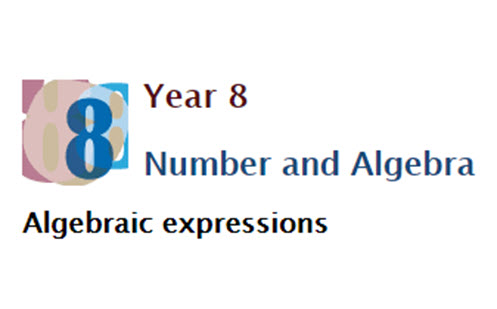Go to website

# Algebraic expressions

This resource provides the teacher with detailed explanations and worked examples on the following areas of algebraic expression: distributive law, using strategies such as the area model; recognising the relationship between factorising and expanding; and understanding the laws used with numbers can also be used with algebra. Students can access the resource to deepen their understanding of the topic as they read through the worked examples and complete the practice questions presented.

Year level(s) Year 8
Audience Student, Teacher
Purpose Content knowledge, Evidence-based approaches, Student task, Teaching resource, Teaching strategies
Teaching strategies and pedagogical approaches Explicit teaching, Collaborative learning, Worked examples
Keywords distributive law, four operations, expanding brackets, factorising, numerical factors, common factor, expansion, simplifying, pronumerals, commutative and associative laws, difference

## Curriculum alignment

Curriculum connections Numeracy
Strand and focus Algebra, Number, Apply understanding, Build understanding
Topics Algebraic expressions, Multiplication and division, Addition and subtraction, Multiples, factors and powers
AC: Mathematics content descriptions
ACMNA190 Extend and apply the distributive law to the expansion of algebraic expressions
ACMNA191 Factorise algebraic expressions by identifying numerical factors
ACMNA192 Simplify algebraic expressions involving the four operations
National numeracy learning progression Number patterns and algebraic thinking - NPA8
Multiplicative strategies